## Dynamic Content

Use the Dynamic Content feature to generate variations of the same question

Dynamic Content is a feature that allows you to define variables and dynamically use those variables in your question. This means that you can randomly deliver multiple variations of the same question to your students. Dynamic Content can be used in ANY question type and can be in the form of text, LaTeX, or imagery. It is most popularly used in Math and Chemistry, Multiple Choice Questions, Matching, Sort, and Order Lists.

### Example

To help you visualise how Dynamic Content works, let's outline a very simple example.

I have a math question that requires the student to add two numbers together and input the correct result. I want all students to get the same question structure, i.e. 'Add two numbers together', however, I don't want every student to get the same two numbers in their question.

Dynamic Content allows you to define a question structure (Add X + Y) and dynamically select values for X and Y from a list of pre-defined variables. This means that you can deliver different variations of the same question to your students using pre-defined data.

With the Dynamic Content feature, you don't need to worry about manually entering large amounts of data. Data can be directly imported from a CSV.

There are two steps to setting up Dynamic Content.
2. Using Dynamic Content when writing your question

1. To begin, go to the exam editor and create a new question. Select 'settings' on the top right of the question.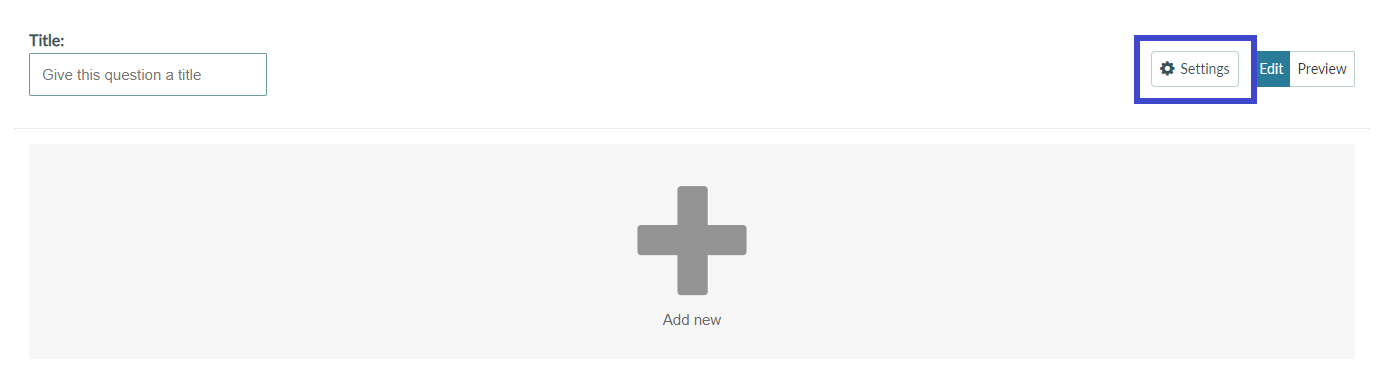2. Select the 'Data table' tab.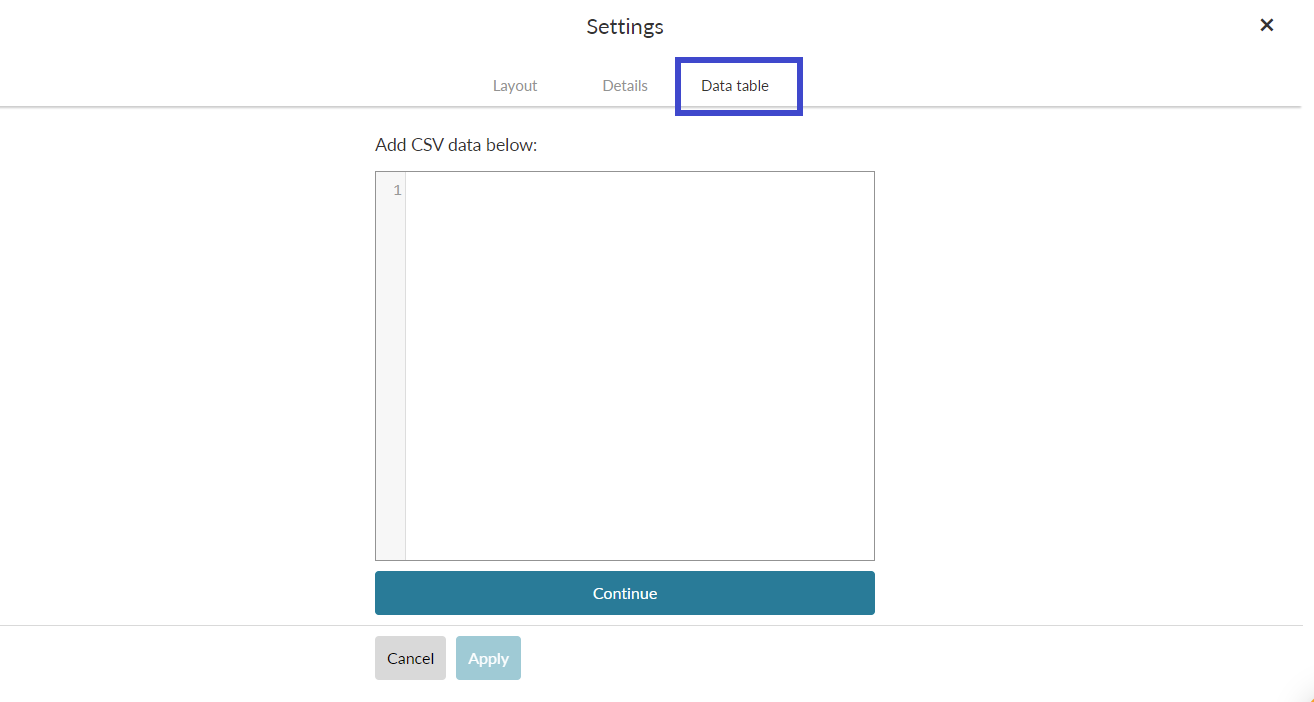3. This is the section where you define your variables. The data entered here is converted from CSV format into a data table.

Data should take the following format:

HEADER: Variable 1, Variable 2, Variable 3,
ROW 1: Value 1, Value 2, Value 3
ROW 2: Value 1, Value 2, Value 3

The first row should contain the variable names. Then each row after that is considered a variant and should contain the value for each variable in that variant.

Let's take a look at a practical example in a spreadsheet. In this example, I will define three variations for an addition question.In my spreadsheet, I have defined my three variables (Operand 1, Operand 2, Result). Then below, each row contains a different value for each variable. Given the value I have entered in the result column, I would expect that:

Operand 1 + Operand 2 = Result

4. To use this data to build variations of a question, simply save your file as a '.CSV', and drag and drop it into the text area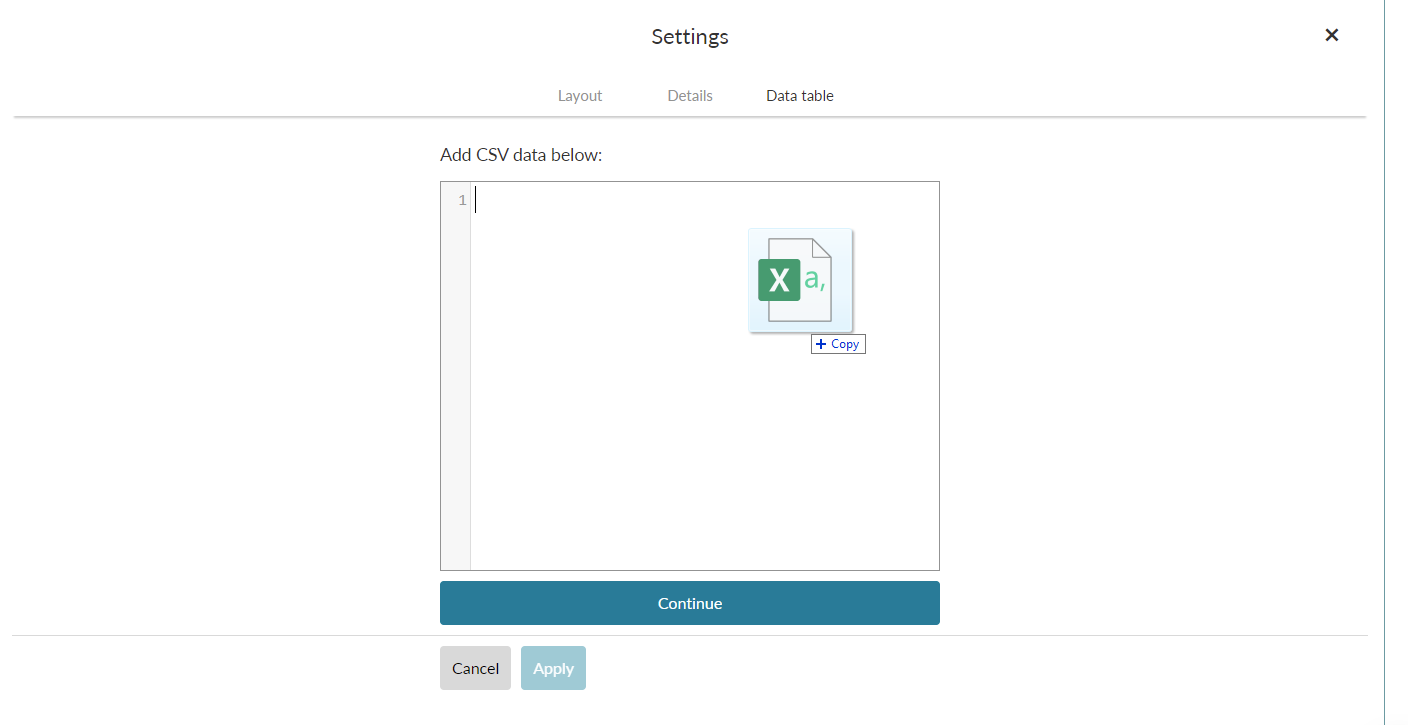5. You should then see your content displayed in the text area as shown below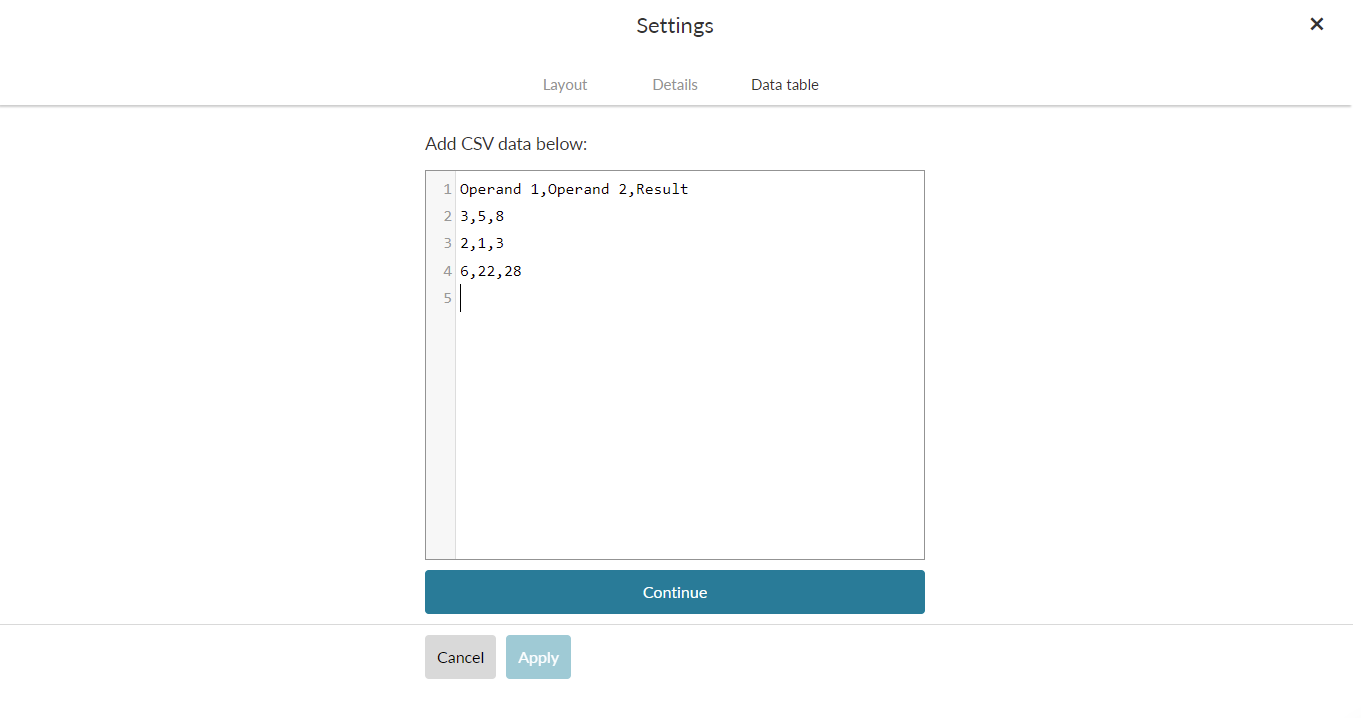6. Click 'Continue' and your data will be transformed into a data table as below. Click 'Apply' to add the Dynamic Content to your question.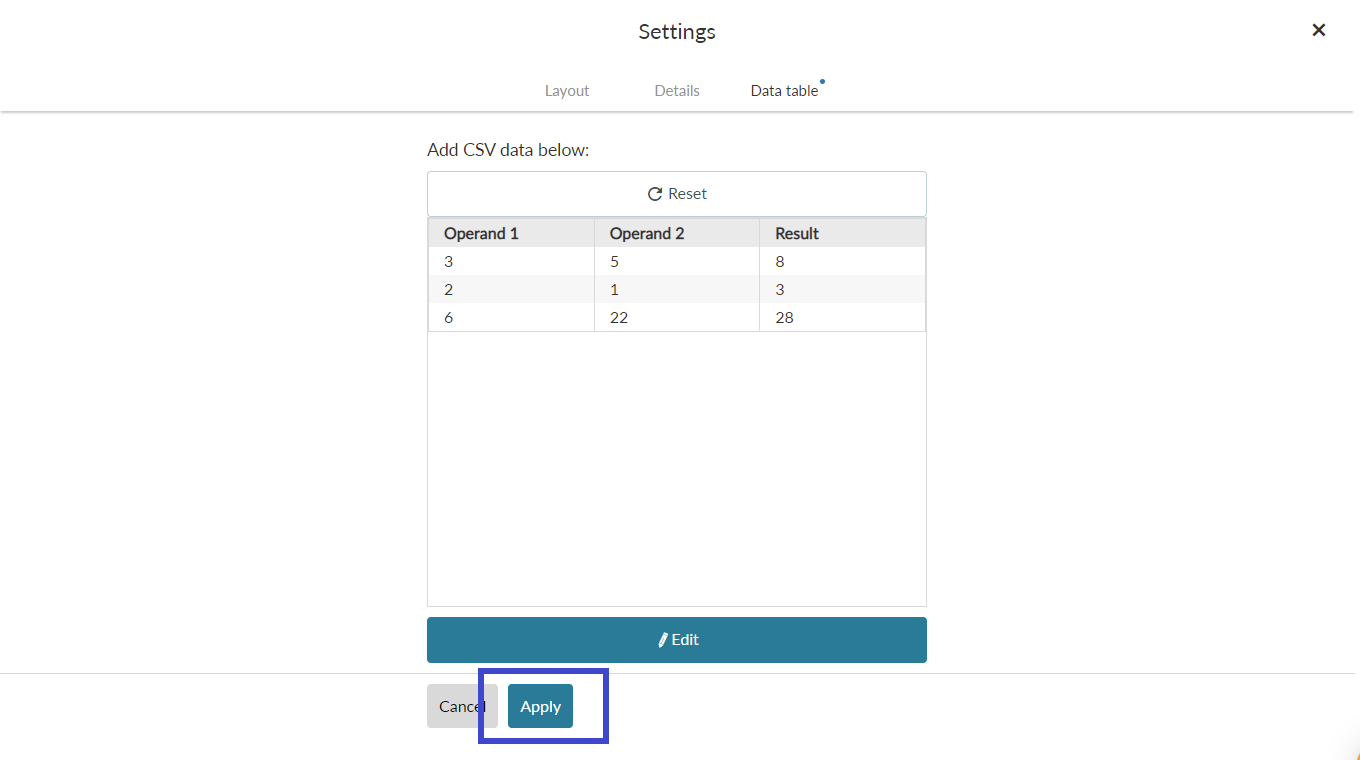## Using Dynamic Content when writing your question

Now that we have added the content to our question, we can make use of those variables to create variants of the same question.

For this example, we are going to use a Cloze Math question type.

1. Create a new Cloze Math question. In the Compose question section type 'Fill in the blank'.

2. Remove all pre-filled content from the formula template section, then click on the 'Response' icon in the text tools to add a response box3. You should now see Response 1 inside the set correct answers section. At this stage, you can also set the points you want to award for a correct answer. For this example, I will leave it as 1 point. The next step is to click on Response 1 to expand it.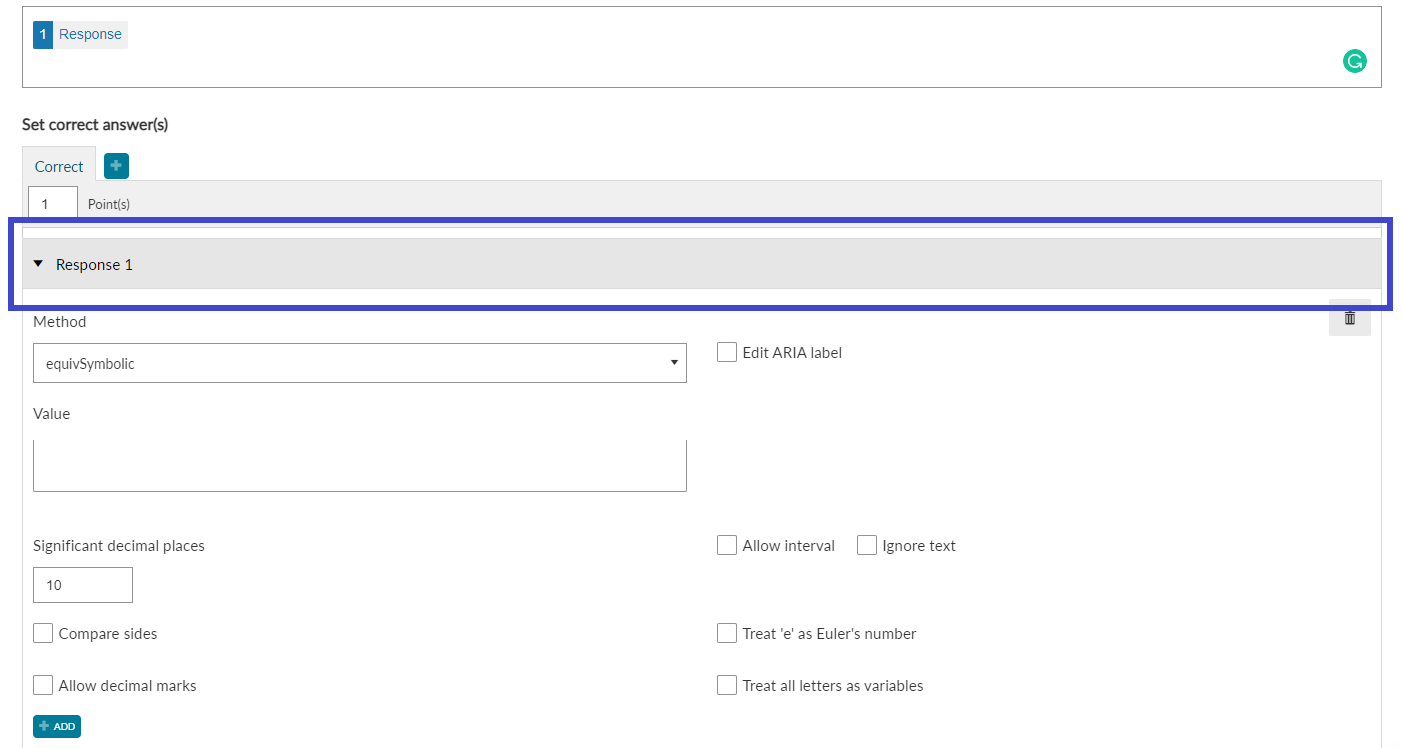4. Inside the 'Method' dropdown, select 'equivSymbolic'.  Then click on the value box to begin defining how the correct result will be calculated. In this case, the correct result is when  'Operand 1' + 'Operand 2' equals 'Result'. Select the Dynamic Content Icon and select 'Operand 1' to begin building the formula as shown below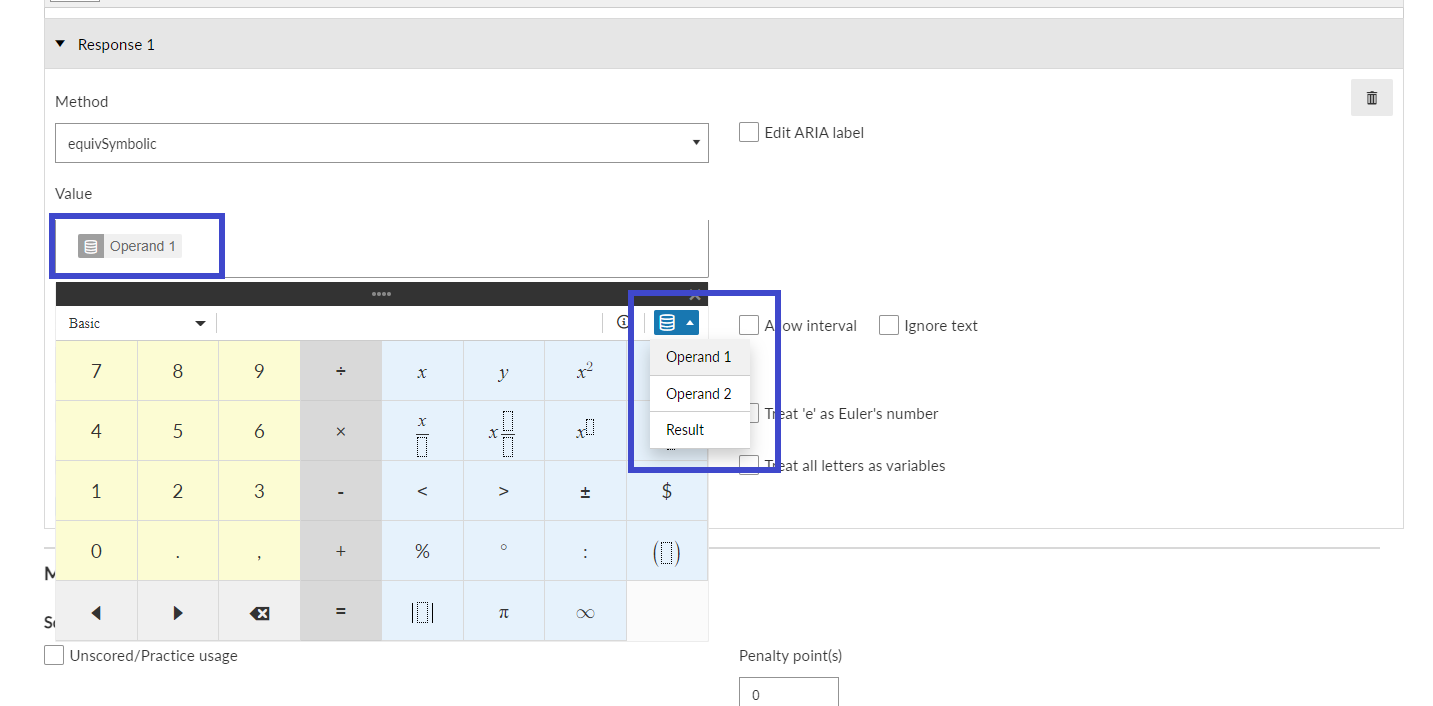5. Then add a '+', then add 'Operand 2' from the Dynamic Content menu, then an '=', and finally add Result. When complete it should look like this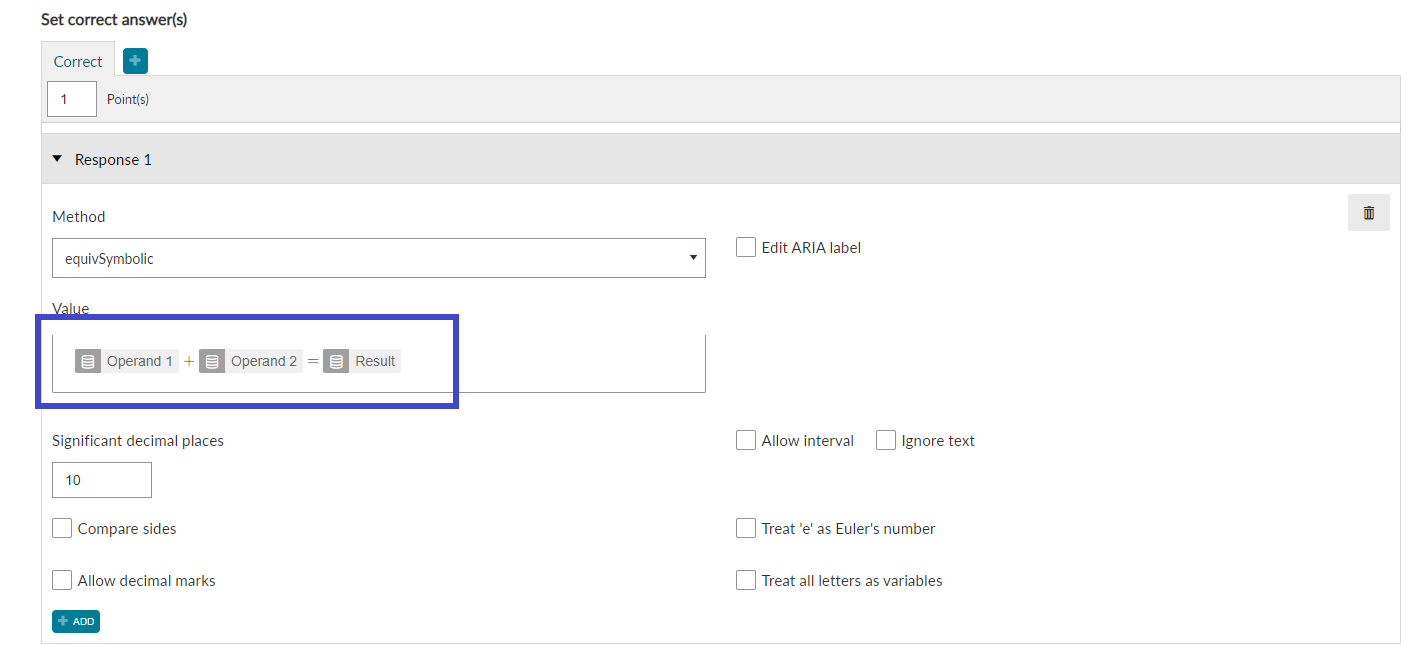6. The final step is to define the layout of our question. In this case, we want our question to be in the form of Operand 1 + Operand 2 = [Enter Response]

To achieve this, scroll down to the Layout section, and under the Response Container (global), select the formula template text box to open up the formula creator.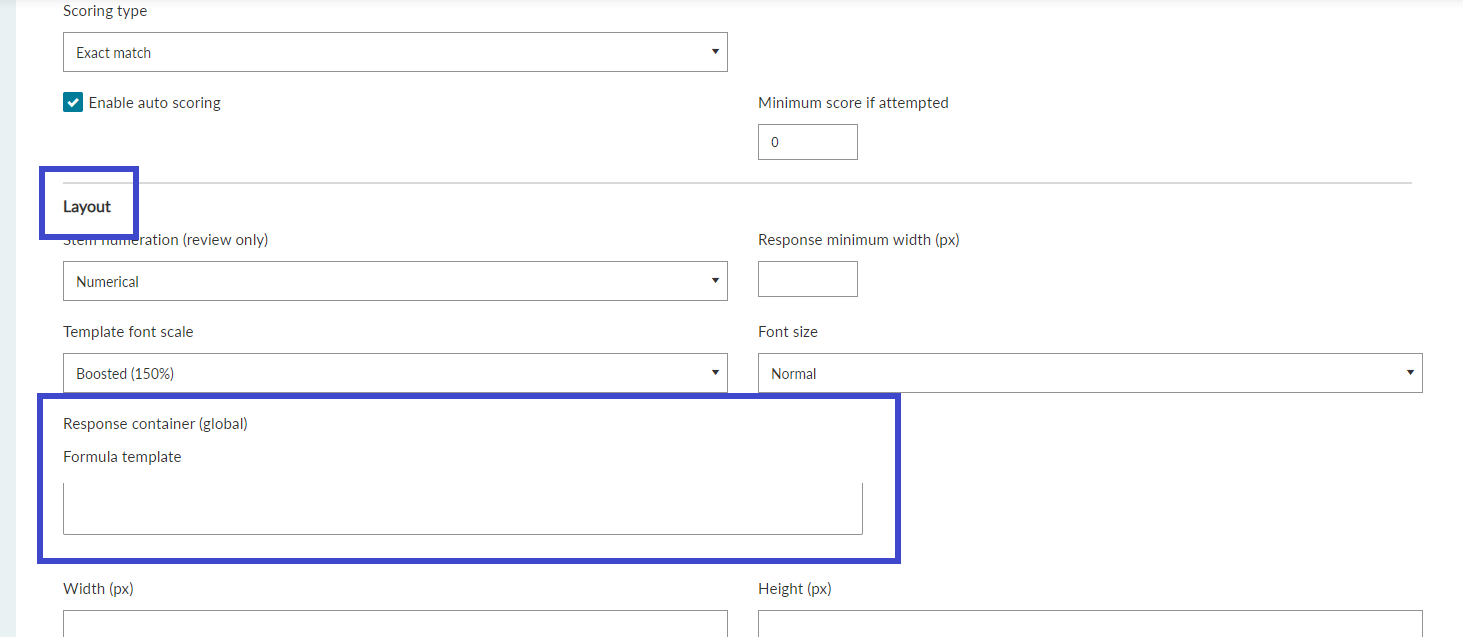7. Similar to the setup for the response formula, we define the formula using the Dynamic Content except we replace 'Result' with a 'Response block' as shown below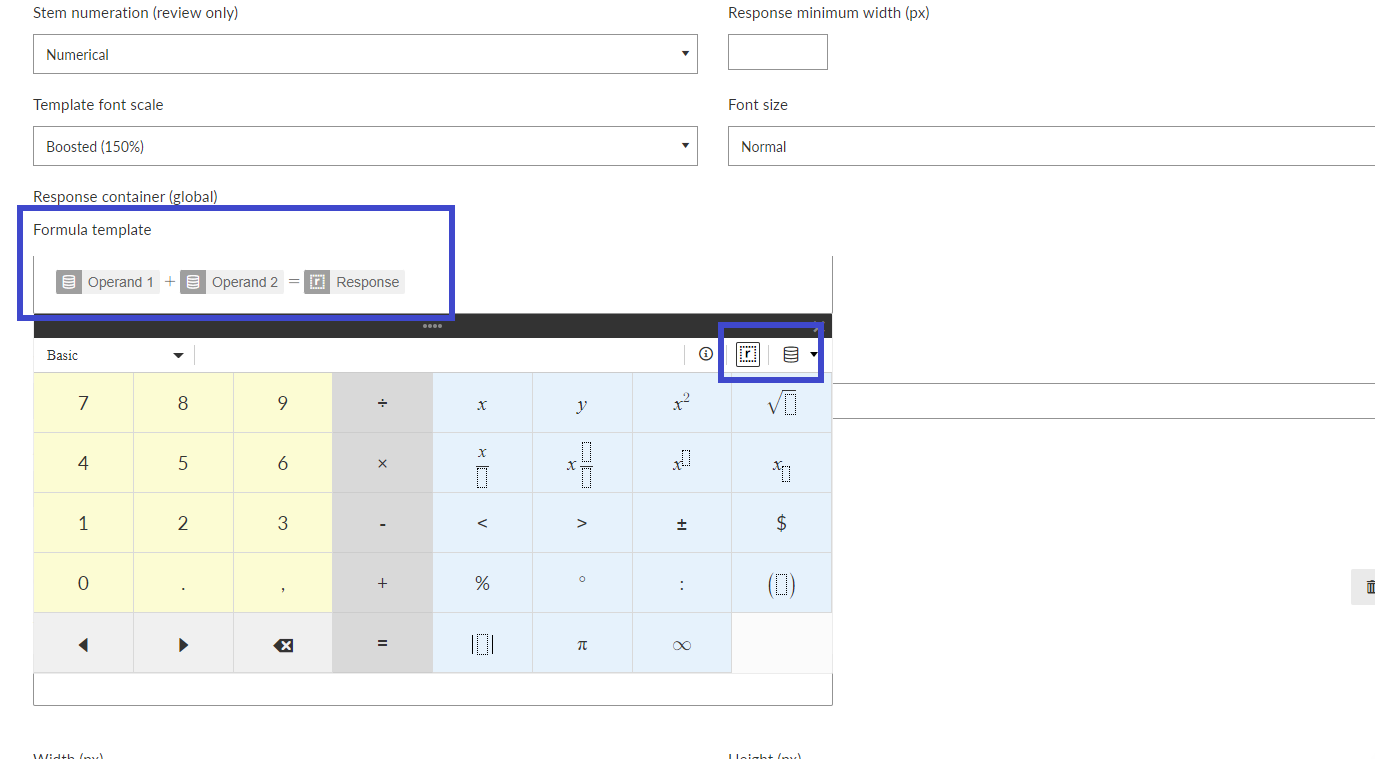8. That is it. We have defined the structure of our question and the formula for validating the correct response.

Students will be presented with a question like the one shown below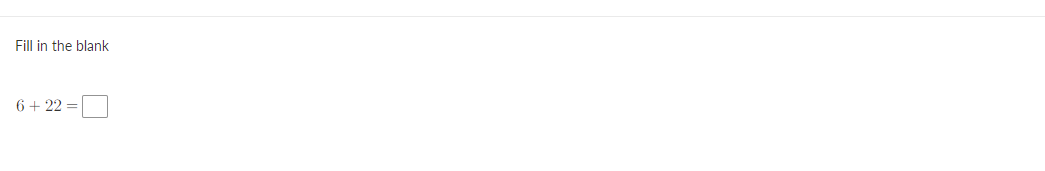Every student that takes the exam will now be given a dynamically generated variation of the question chosen at random. If you want to add more question variants, all you need to do is add an extra row to your Dynamic Content and the rest will be taken care of.